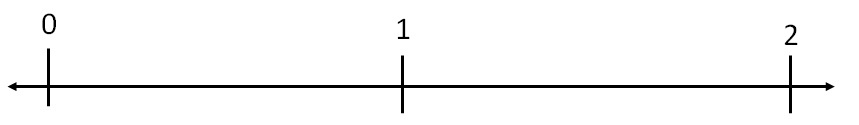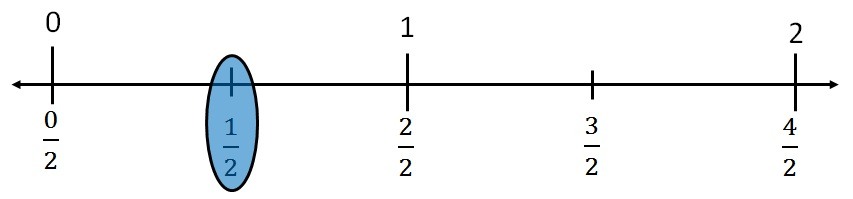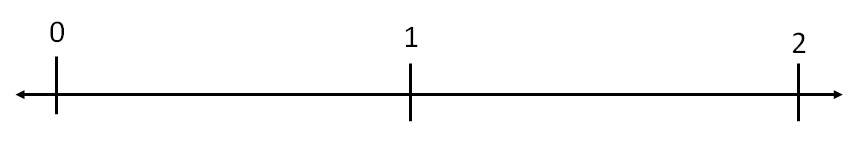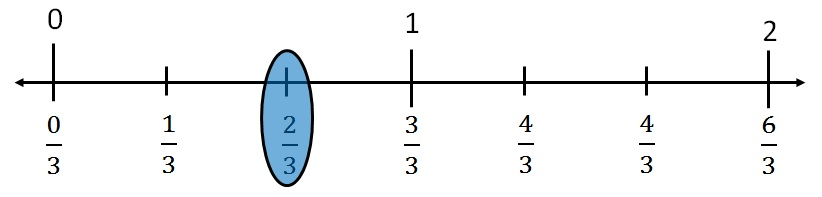#### Draw 1/2 on number line

Let’s us draw number line from 0 to 2Since in 1/2, we have denominator 2.

We divide line between 0 & 1 into 2 equal parts#### Draw  2/3 on number line

Let’s us draw number line from 0 to 2Since in 2/3, we have denominator 3.

We divide line between 0 & 1 into 3 equal parts1. Chapter 7 Class 6 Fractions
2. Concept wise
3. Fractions on the number line